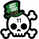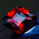71026 visningar
Simple indicator to visualize Transient Zones (TZ) and Potential Transient Zones (PTZ).
The last 3 numbers in the header represent: probability of true TZ (black), probability of PTZ (navy) and probability of PTZ resolving (gray).

Version 1.1:
- Added TZ/PTZ occurrence probability thanks to SPYderCrusher

Todo:
- Mid bar transient zones detection
- Draw rectangles for zones (once rectangle drawing gets implemented in pine script) or use dot/circle lines
- Mark forming PTZs more clearly

Previous version 1.0:
```//By Jurij w/ TZ percent occurrence by SPYderCrusher
study("Transient Zones v1.1", "TZ", overlay=true)

//inputs
h_left = input(title="H left", type=integer, defval=10)
h_right = input(title="H right", type=integer, defval=10)
sample_period = input(title="Sample bars for % TZ", type=integer, defval=5000)
show_ptz = input(title="Show PTZ", type=bool, defval=true)
show_channel = input(title="Show channel", type=bool, defval=true)

//barCount = nz(barCount) + 1
//check history and realtime PTZ
h_left_low = lowest(h_left)
h_left_high = highest(h_left)
newlow = low <= h_left_low
newhigh = high >= h_left_high
plotshape(newlow and show_ptz, style=shape.triangledown, location=location.belowbar, color=red)
plotshape(newhigh and show_ptz, style=shape.triangleup, location=location.abovebar, color=green)
channel_high = plot(show_channel ? h_left_low : 0, color=silver)
channel_low = plot (show_channel ? h_left_high : 0, color=silver)

//check true TZ back in history
central_bar_low = low[h_right + 1]
central_bar_high = high[h_right + 1]
full_zone_low = lowest(h_left + h_right + 1)
full_zone_high = highest(h_left + h_right + 1)
central_bar_is_highest = central_bar_high >= full_zone_high
central_bar_is_lowest = central_bar_low <= full_zone_low
plotarrow(central_bar_is_highest ? -1 : 0, offset=-h_right-1)
plotarrow(central_bar_is_lowest ? 1 : 0, offset=-h_right-1)

//TZ probability calc
x = central_bar_is_highest ? 1 : 0
high_bar_tz_count = cum(x)

y = central_bar_is_lowest ? 1 : 0
low_bar_tz_count = cum(y)

total_tz = high_bar_tz_count + low_bar_tz_count
percent_tz_high = (high_bar_tz_count / sample_period) * 100
//plot(percent_tz_high, color = lime, transp=100)
percent_tz_low = (low_bar_tz_count / sample_period) * 100
//plot(low_bar_tz_count, color=red, transp=100)
percent_total_tz = (percent_tz_high + percent_tz_low)
plot(percent_total_tz, color=black, transp=100)

//PTZ probability calc
i = newhigh ? 1 : 0
high_bar_ptz_count = cum(i)

j = newlow ? 1 : 0
low_bar_ptz_count = cum(j)

total_ptz = high_bar_ptz_count + low_bar_ptz_count
percent_ptz_high = (high_bar_ptz_count / sample_period) * 100
//plot(percent_ptz_high, color=green, transp=100)
percent_ptz_low = (low_bar_ptz_count / sample_period) * 100
//plot(percent_ptz_low, color=maroon, transp=100)
percent_total_ptz = (percent_ptz_high + percent_ptz_low)
plot(percent_total_ptz, color=navy,  transp=100)

//PTZ resolving probability calc
percent_ptz_resolved = (1 - (total_tz / total_ptz)) * 100
plot(percent_ptz_resolved, color=gray,  transp=100)
```

## KommentarerIs there a way to add an alert to the Red and Green arrows?
Svarayrainville
@yrainville, Is there an actual script with Transient that actually works for trading all time frames?
Svara